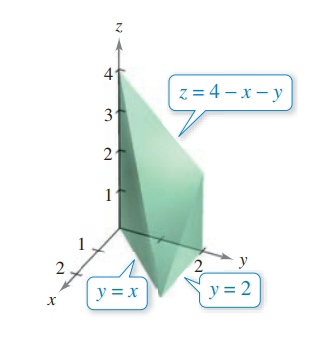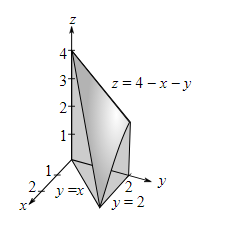Chapter 7.9, Problem 17E### Calculus: An Applied Approach (Min...

10th Edition
Ron Larson
ISBN: 9781305860919

#### Solutions

Chapter
Section### Calculus: An Applied Approach (Min...

10th Edition
Ron Larson
ISBN: 9781305860919
Textbook Problem
1 views

# Finding the Volume of a Solid Region In Exercises 13-20, use a double integral to find the volume of the solid region.To determine

To calculate: The volume of solid regionExplanation

Given information:

The provided solid region is

Formula used:

The procedure to calculate volume of surface z=f(x,y),

Step-1: Write the equation of surface in the form z=f(x,y)

Step-2: Sketch the projected region R in the x-y-plane.

Step-3: Determine the order of limits of integration.

Step-4: Evaluate the volume of solid region,

Volume=mnabf(x,y)dxdy

Here, the projected region is R:

myn and axb

Calculation:

Consider graph of solid region,

The equation of upper surface written as follow.

z=4xy

The following table shown different coordinate of (x,y) for y=x.

 x-Coordinate y-Coordinate (x,y) Coordinate 0 0 (0,0) 2 2 (2,2)

The projected region R: 0xy,0y2 in the x-y plane written as follow,

The bounds for x are 0xy and bounds for y are 0y2.

The volume for the solid region is,

Volume=020y(4xy)dxdy

Now integrate with respect to x and apply the limit

### Still sussing out bartleby?

Check out a sample textbook solution.

See a sample solution

#### The Solution to Your Study Problems

Bartleby provides explanations to thousands of textbook problems written by our experts, many with advanced degrees!

Get Started

#### Expand each expression in Exercises 122. (x22x+1)2

Finite Mathematics and Applied Calculus (MindTap Course List)

#### In Exercises 43-46, find f(a + h) f(a) for each function. Simplify yoiir answer. 45. f(x) = 4 x2

Applied Calculus for the Managerial, Life, and Social Sciences: A Brief Approach

#### In problems 23-58, perform the indicated operations and simplify. (x3+5x26)(x22)

Mathematical Applications for the Management, Life, and Social Sciences

#### The area of the shaded region is given by:

Study Guide for Stewart's Multivariable Calculus, 8th